# How to change the order of bars in bar chart in R ?

• Last Updated : 29 Jun, 2021

In this article, we will discuss how to change the order of bars in bar chart in R programming language. We can change the order of bars by using two plots ggplot and barplot.

### Method 1: Ggplot re-ordering

Firstly create a sample dataset and plot the graph-Manual. Now let’s reorder them accordingly.

Dataset in Use: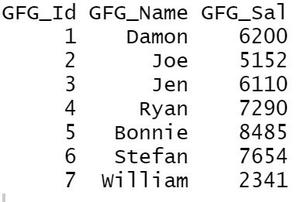Employee Salary Details

Reordering in ggplot is done using theme() function. Within this, we use axis.text.x with the appropriate value to re-order accordingly. By default, geom_bar uses stat=”bin”. This makes the height of each bar equal to the number of cases in each group,  If you want the heights of the bars to represent values in the data, use stat=”identity” and map a value to the y aesthetic.

• geom_bar() : Use for the Bar charts

Syntax:

geom_bar( mapping = NULL, data = NULL, stat = “count”, color=’blue’; fill=’yellow’ width = NULL, na.rm = FALSE, orientation = NA, show.legend = NA,…,)

• Theme: Themes can be used to give plots a consistent customized look.

Syntax:

theme( line, rect, text, title, aspect.ratio, axis.text.x, axis.text.x.top, axis.text.x.bottom,)

Parameter:

• line  : all line elements (element_line())
• rect  :all rectangular elements (element_rect())
• text  : all text elements (element_text())
• title  :all title elements: plot, axes, legends (element_text(); inherits from text)
• aspect.ratio  :aspect ratio of the panel.
• axis.text.x : labels along with axes (element_text()). Specify all axis labels (axis.text), labels

### Manually Order

To reorder bars manually, you have to pass stat=”identity” in the geom_bar() function.

Example:

## R

 `library``(ggplot2)`` ` `# Create the data frame.``gfg.data <- ``data.frame``(``  ``GFG_Id = ``c ``(1:7), ``  ``GFG_Name = ``c``(``"Damon"``,``"Joe"``,``"Jen"``,``"Ryan"``,``"Bonnie"``,``"Stefan"``,``"William"``),``  ``GFG_Sal = ``c``(6200,5152,6110,7290,8485,7654,2341))``print``(gfg.data)`` ` `# GGPLOT``x <- ``ggplot``(gfg.data, ``aes``(x = GFG_Name, y = GFG_Sal))`` ` `x <- x + ``geom_bar``(stat=``"identity"``, color=``'lightgreen'``,fill=``'lightgreen'``)`` ` `x <- x + ``theme``(axis.text.x=``element_text``(angle=45, hjust=0.9))``x`

Output: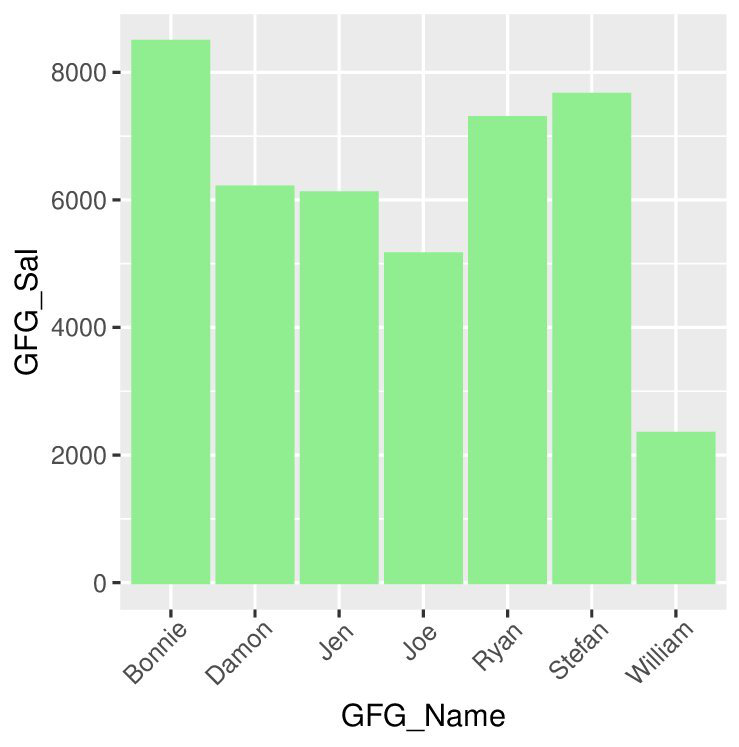### Re-ordering the in ascending and descending order

The reorder function is used here to change the order of the graphs.

Syntax :

ggplot(dataframe name, aes(x=reorder(column1,±column2),y=column2)

Here if you want ascending order then you’ll use ‘+’ plus sign, if you want in descending order then you should use ‘-‘ minus sign.

Note: Column2 must be the column with numeric data.

Example: Let us first show the same bar plot in ascending order.

## R

 `library``(ggplot2)`` ` `# Create the data frame.``gfg.data <- ``data.frame``(``  ``GFG_Id = ``c ``(1:7), ``  ``GFG_Name = ``c``(``"Damon"``,``"Joe"``,``"Jen"``,``"Ryan"``,``"Bonnie"``,``"Stefan"``,``"William"``),``  ``GFG_Sal = ``c``(6200,5152,6110,7290,8485,7654,2341))`` ` `print``(gfg.data)`` ` `# GGPLOT``x <- ``ggplot``(gfg.data, ``aes``(x = ``reorder``(GFG_Name, +GFG_Sal), y = GFG_Sal))`` ` `x <- x + ``geom_bar``(stat=``"identity"``, color=``'red'``,fill=``'red'``)`` ` `x <- x + ``theme``(axis.text.x=``element_text``(angle=45, hjust=0.9))``x`

Output: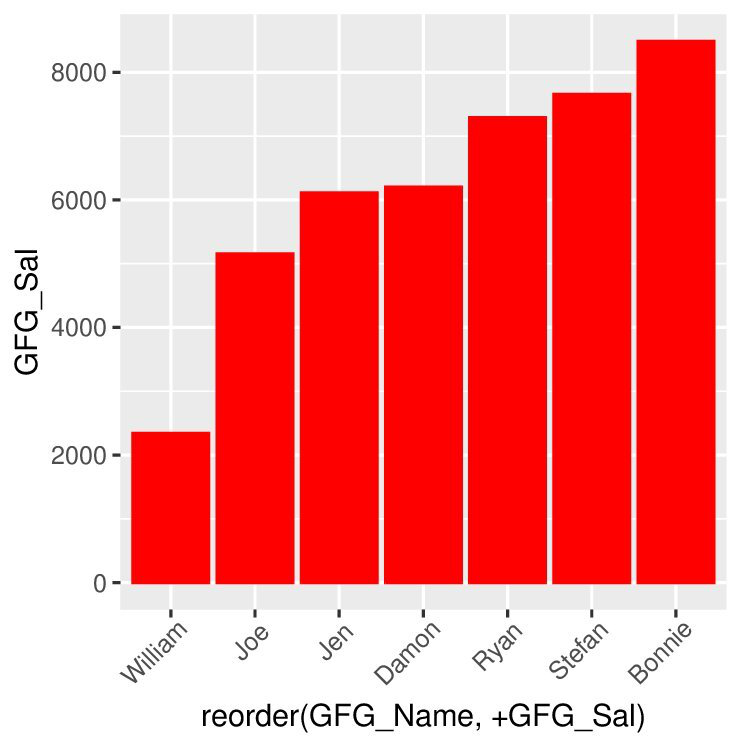Example: Now let’s look at descending ordered plot

## R

 `library``(ggplot2)`` ` `# Create the data frame.``gfg.data <- ``data.frame``(``  ``GFG_Id = ``c ``(1:7), ``  ``GFG_Name = ``c``(``"Damon"``,``"Joe"``,``"Jen"``,``"Ryan"``,``"Bonnie"``,``"Stefan"``,``"William"``),``  ``GFG_Sal = ``c``(6200,5152,6110,7290,8485,7654,2341))`` ` `print``(gfg.data)`` ` `# GGPLOT``x <- ``ggplot``(gfg.data, ``aes``(x = ``reorder``(GFG_Name, -GFG_Sal), y = GFG_Sal))`` ` `x <- x + ``geom_bar``(stat=``"identity"``, color=``'violet'``,fill=``'violet'``)`` ` `x <- x + ``theme``(axis.text.x=``element_text``(angle=45, hjust=0.9))``x`

Output: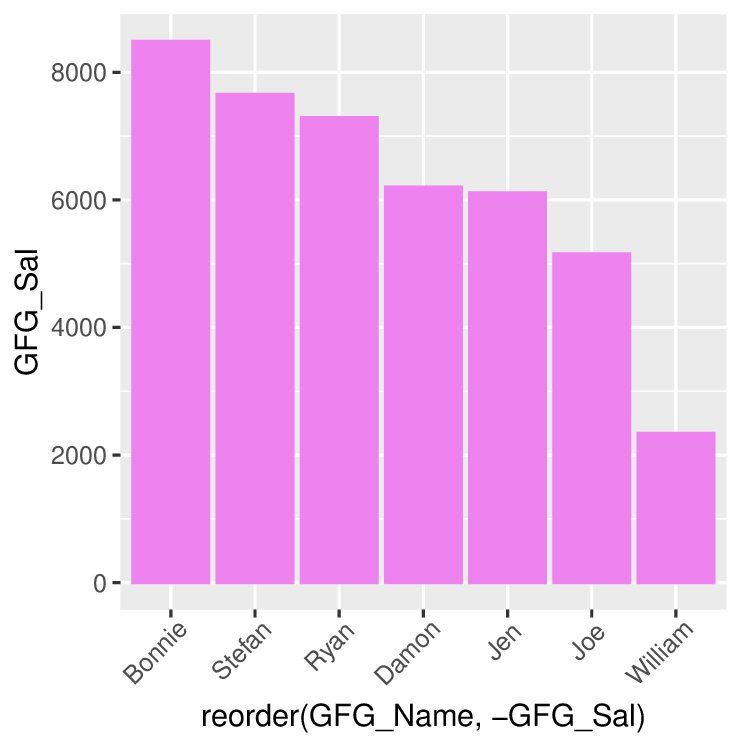### Method 2: Using Barplot re-ordering

Firstly create a sample dataset and plot the graph. Now let us look at the reordering of the bars.

Dataframe in use: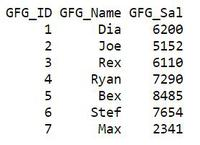In R, barplot() function

Syntax:

barplot(height, name.args = NULL, col = NULL, main = NULL)

Parameter:

• height: You can specify either a Vector or a Matrix of values.
• name.args :a Vector of names you want to plot below each bar or group of bars in an R bar chart.

### Manually Ordering

For manual ordering pass the order to the x-axis while creating the dataframe.

Example:

## R

 `# Create the data for the chart``GFG_ID   <- ``c``(1:7)``GFG_Sal  <- ``c``(6200,5152,6110,7290,8485,7654,2341)``GFG_Name <- ``c``(``"Dia"``,``"Joe"``,``"Rex"``,``"Ryan"``,``"Bex"``,``"Stef"``,``"Max"``)``X <- ``data.frame``(GFG_ID,GFG_Name,GFG_Sal)``X`` ` `# Give the chart file a name``png``(file = ``"barchart_months_salary.png"``)`` ` `# Plot the bar chart ``barplot``(GFG_Sal,names.arg=GFG_Name,xlab=``"Employee Name"``,``        ``ylab=``"Salary Range"``,col=``"black"``,``main=``"Salary chart"``,border=``"Red"``)`` ` `# Save the file``dev.off``()`

Output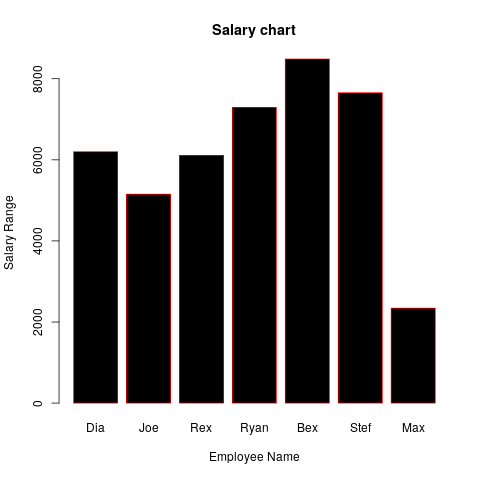### Re-ordering the graph in ascending and descending order

The function used here is the order (order returns a permutation that rearranges its first argument into ascending or descending order)

Syntax –

order(…, na.last = TRUE, decreasing = FALSE)

Parameter:

na.last: for controlling the treatment of NAs

Let us first look at the ascending ordered plot.

Example:

## R

 `GFG_ID  <- (``LETTERS``[1:7])``GFG_Sal  <- ``c``(6200,5152,6110,7290,8485,7654,2341)`` ` `data <- ``data.frame``(GFG_Sal, GFG_ID)`` ` `barplot``(data[``order``(data[,1],decreasing=``FALSE``),``        ``][,1],names.arg=data[``order``(data[,1],decreasing=``FALSE``),]``        ``[,2],col=``"blue"``,xlab=``"Employee Name Initial Letter"``,``        ``ylab=``"Salary Range"``,main=``"Salary chart"``,border=``"black"``)`

Output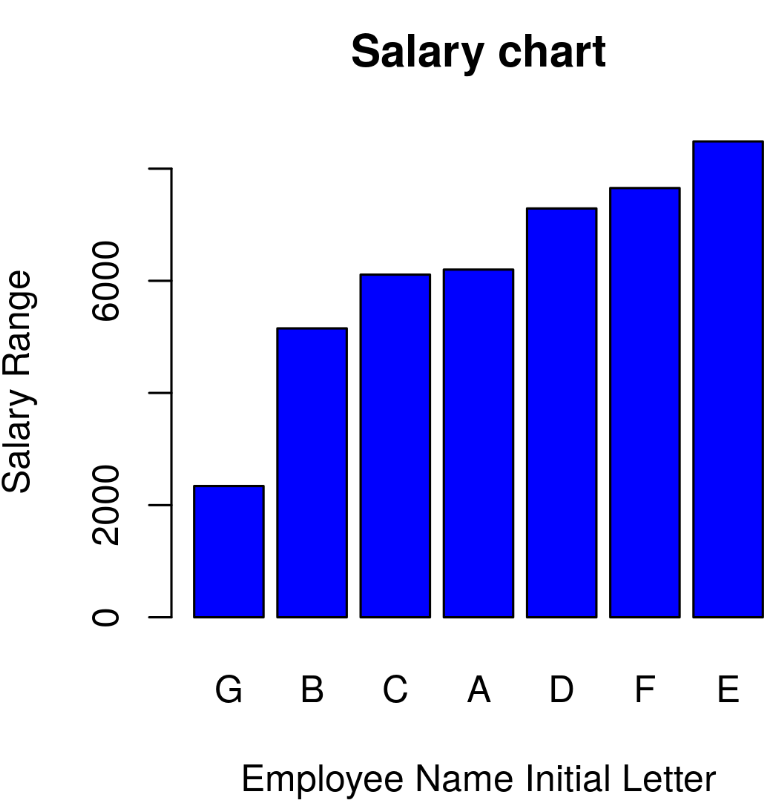Now let us look at the descending ordered plot.

Example:

## R

 `GFG_ID  <- (``LETTERS``[1:7])``GFG_Sal  <- ``c``(6200,5152,6110,7290,8485,7654,2341)`` ` `data <- ``data.frame``(GFG_Sal, GFG_ID)`` ` `barplot``(data[``order``(data[,1],decreasing=``TRUE``),``        ``][,1],names.arg=data[``order``(data[,1],decreasing=``TRUE``),``        ``][,2],col=``"blue"``,xlab=``"Employee Name Initial Letter"``,``        ``ylab=``"Salary Range"``,main=``"Salary chart"``,border=``"black"``)`

Output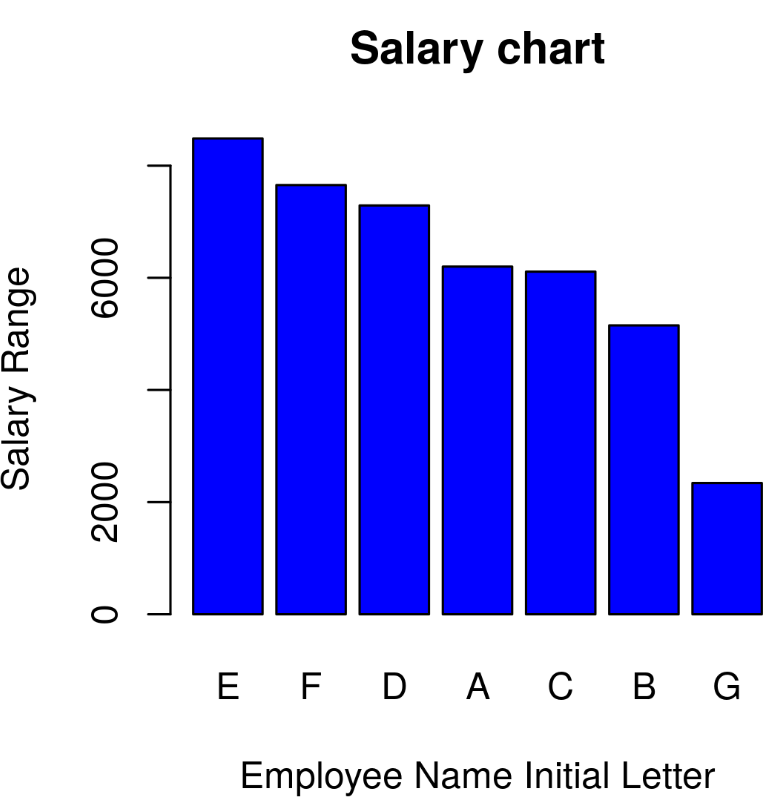My Personal Notes arrow_drop_up